### Home > A2C > Chapter Ch9 > Lesson 9.2.2 > Problem9-91

9-91.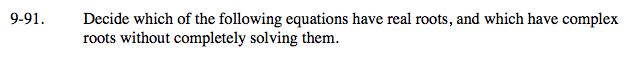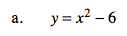Find the discriminant.

b2 − 4ac = 02 − 4(1)(−6) = 24

This equation has real roots.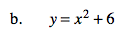b2 − 4ac = 02 − 4(1)(6) = −24

This equation has complex roots.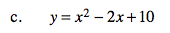See part (b).

This equation has complex roots.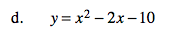See part (a).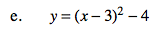Find the vertex.
Is it above or below the x-axis?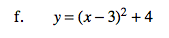See part (e).